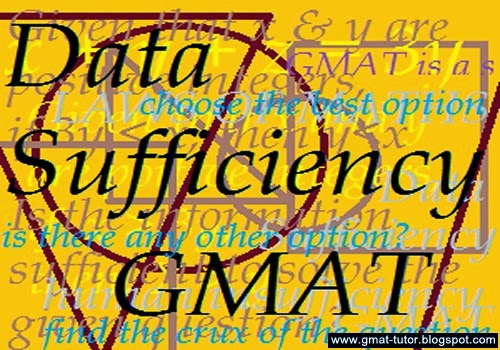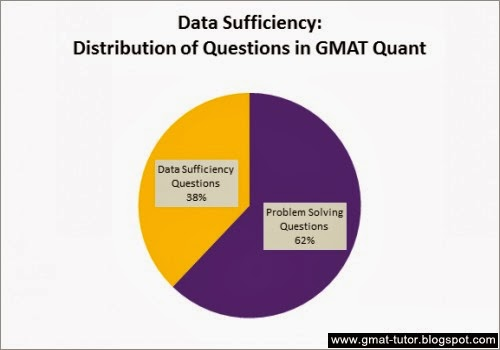## Sunday, January 12, 2014

### GMAT Data Sufficiency Practice Questions

In this post, I am giving you some data sufficiency practice questions. At the end of all the questions, answers with the explanations are written. It is suggested don’t look at the answers before answering all the questions. In each of the problems, a question is followed by two statements containing certain data. You are to find out whether the data provided by the statements is sufficient to answer the question.Answer Choices

A.    If statement (1) and (2) taken together are not sufficient to answer the question, requiring more data pertaining to the problem.
B.    If statement (1) and (2) taken together are sufficient to answer the question, even though neither statement by itself is sufficient;
C.    If statement (1) by itself is sufficient to answer the question, but statement (2) by itself is not;
D.    If either statement by itself is sufficient to answer the question;
E.    If statement (2) by itself is sufficient to answer the question, but statement (1) by itself is not;

Question no: 1     A shopper bought a dress pent and a shirt during a sale. Which item did she purchase at the greater dollar value?
(1)    She bought the dress pent at 15 percent discount.
(2)    She bought the shirt at 20 percent discount.
A.
B.
C.
D.
E.

Question no: 2     If she did not stop along the way, what speed did bill average on her 3- hour trip?
(1)    She traveled a total of 120 miles.
(2)    She traveled the half distance at 30 miles per hour, and half the distance at 60 miles per hour.
A.
B.
C.
D.

Question no: 3     Does a=b?
(1)    a2-b2 =0.
(2)    (a-b)2 =0.
A.
B.
C.
D.

Question no: 4     Does a+b positive?
(1)    a-b is positive.
(2)    b-a is positive.
A.
B.
C.
D.

Question no: 5     If X is an integer, is X evenly divisible by 3?
(1)    2X is evenly divisible by 3.
(2)    3X is evenly divisible by 3.
A.
B.
C.
D.ANSWERS AND EXPLANATIONS

Question no: 1 (E)
(1)    Insufficient: Only information about the dress pent is given. We know nothing about the shirt.
(2)    Insufficient: Only information about the shirt is given. We know nothing about the dress pent.
All we can determine is that greater percentage discount was obtained on the dress shirt. Whether this translates into a greater dollar discount cannot be determined.

Question no: 2 (D)
(1)    Sufficient: With the distance known, we could plug it into the rate formula and computer bill’s rate.
(2)    Sufficient: If she covered the same distance at 30 mph as she did at 60 mph, she must have been traveling at 30 mph for twice as long as she was at 60 mph. Given that she traveled for 3 hours, she traveled at 30 mph for 2 hours and 60 mph for 1 hour. That comes to 120 miles total distance, and again we solve for the rate.
All we can determine is that greater percentage discount was obtained on the dress shirt. Whether this translates into a greater dollar discount cannot be determined.

Question no: 3 (B)
(1)    Insufficient: It may look like the two are equal, but not necessarily. All the statement tells us is that a2 is equal to b2 . That does not mean that a equals b, because one could be negative and other positive.
(2)    Sufficient: This tells us that (a-b) (b-a) =0. So, (a-b) =0. The only way the difference between the two variables can be 0 is if they are the same.

Question no: 4 (E)
(1)    Insufficient: Pick 10 for a and 5 for b. This satisfies the statement and would allow us the answer “YES” the question. We can’t stop here through; we have to try different values to see if we can answer the question, “NO”. Try 5 for a and -10 for b. These values satisfy statement (1) but allow us to answer the question “no”.
(2)    Insufficient: Try the same values. Those values allow us to answer “no” to the question. But we need to consider other values. If we set b equal to -5 and a equal to 10, we can answer “yes” to the question.
You can guess between ( C ) and (E ) or you could plug in some more members. As it turn out the two statements are equivalent.  So they are just as insufficient together as they are separate.

Question no: 5 (A)
(1)    Sufficient: Since the quantity 2X is divisible by 3, one of those two factors must be divisible by 3. Since 1 isn’t; X must be.
(2)    Insufficient: We know the quantity 3X is evenly divisible by 3, which means that at least one of the factors must be divisible by 3. The problem, through, is that 3 is evenly divisible by 3, making it impossible for us to determine if X is.